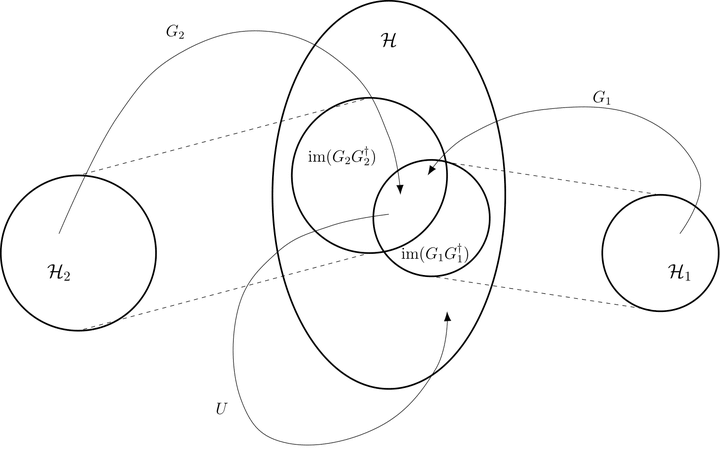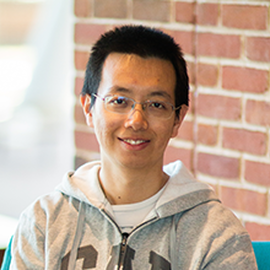# Quantum singular value transformation and beyond: exponential improvements for quantum matrix arithmetics### Abstract

Quantum computing is powerful because unitary operators describing the time-evolution of a quantum system have exponential size in terms of the number of qubits present in the system. We develop a new “Quantum singular value transformation” algorithm capable of harnessing this exponential advantage, that can apply polynomial transformations to the singular values of a block of a unitary, generalizing the optimal Hamiltonian simulation results of Low and Chuang.The proposed quantum circuits have a very simple structure, often give rise to optimal algorithms and have appealing constant factors, while typically only use a constant number of ancilla qubits.

We show that singular value transformation leads to novel algorithms. We give an efficient solution to a “non-commutative measurement problem” used for efficient ground-state-preparation of certain local Hamiltonians, and propose a new method for singular value estimation. We also show how to exponentially improve the complexity of implementing fractional queries to unitaries with a gapped spectrum. Finally, as a quantum machine learning application we show how to efficiently implement principal component regression.

“Quantum singular value transformation” is conceptually simple, and leads to a unified framework of quantum algorithms incorporating a variety of quantum speed-ups. Our framework allows describing quantum algorithms on a high level, hopefully making them accessible to researchers even outside the quantum algorithm community. We illustrate this by showing how our meta-algorithm generalizes a number of prominent quantum algorithms, and quickly derive the following algorithms: optimal Hamiltonian simulation, implementing the Moore-Penrose pseudoinverse with exponential precision, fixed-point amplitude amplification, robust oblivious amplitude amplification, fast $\mathsf{QMA}$ amplification, fast quantum OR lemma, certain quantum walk results and several quantum machine learning algorithms.

In order to exploit the strengths of the presented method it, is useful to know its limitations too, therefore we also prove a lower bound on the efficiency of quantum singular value transformation, which often gives optimal bounds.

Type
Publication
In Proceedings of the 51st Annual ACM SIGACT Symposium on Theory of Computing (STOC 2019), pp. 193-204##### Yuan Su
###### Senior Researcher

I work on quantum algorithms for simulating Hamiltonian dynamics. I am particularly interested in the design, analysis, implementation, and application of quantum simulation.